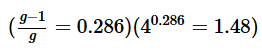Courses

# Test: Strength Of Material- 2

## 25 Questions MCQ Test Mechanical Engineering SSC JE (Technical) | Test: Strength Of Material- 2

Description
This mock test of Test: Strength Of Material- 2 for Mechanical Engineering helps you for every Mechanical Engineering entrance exam. This contains 25 Multiple Choice Questions for Mechanical Engineering Test: Strength Of Material- 2 (mcq) to study with solutions a complete question bank. The solved questions answers in this Test: Strength Of Material- 2 quiz give you a good mix of easy questions and tough questions. Mechanical Engineering students definitely take this Test: Strength Of Material- 2 exercise for a better result in the exam. You can find other Test: Strength Of Material- 2 extra questions, long questions & short questions for Mechanical Engineering on EduRev as well by searching above.
QUESTION: 1

Solution:
QUESTION: 2

Solution:
QUESTION: 3

### The mass flow rate in a refrigeration plant is 2kg/s and its refrigeration effect in 10 kJ/kg. TheRefrigeration capacity of the plant is.

Solution:
QUESTION: 4

The enthalpy in a VCRS at exit of condenser is500 kJ. The refrigeration effect is 1000 kJ. Theenthalpy at the start of compression is

Solution:
QUESTION: 5

In expansion value of a refrigerator the followingproperty remains constant.

Solution:
QUESTION: 6

The type of value used reciprocating refrigerantcompressors is.

Solution:
QUESTION: 7

Which of the following is an Ideal Refrigerationcycle.

Solution:
QUESTION: 8

It the same engine is used as Refrigerator andHeat pump their COPs are relate as:-

Solution:
QUESTION: 9

1 ton of refrigeration is equal to

Solution:
QUESTION: 10

Ice is to be produced using a refrigerator in summer and than in winter. than

Solution:
QUESTION: 11

for a refrigeration cycle the critical point shouldbe

Solution:
QUESTION: 12

At saturated vapour line the value of dryness fraction is

Solution:
QUESTION: 13

In VCRS heat is rejected at

Solution:
QUESTION: 14

Which of the following is disadvantage of wetcompression

Solution:
QUESTION: 15

COP of a cascade Refrigeration system is given as.

Solution:
QUESTION: 16

Which of the following are be used as secondaryrefrigerant.

Solution:
QUESTION: 17

Which of the following group of refrigerant hasozone depletion capability.

Solution:
QUESTION: 18

Which of the following has undesirable critical temperature for indian summer.

Solution:
QUESTION: 19

Which of the following should be property of arefrigerant.

Solution:
QUESTION: 20

For the measurement of quality, the state of the substance is brought from the two-phase region to

Solution:
QUESTION: 21

Sling Psychrometer is used to measure

Solution:
QUESTION: 22

for saturated air, wet bulb depression is

Solution:
QUESTION: 23

During sensible heating specific humidity

Solution:
QUESTION: 24

A Brayton cycle is working under a pressure ratio of 4. it in then reversed to use as an refrigeration
cycle. its cop isSolution:
QUESTION: 25

In psychrometric chart which of the following properties is fixed

Solution: